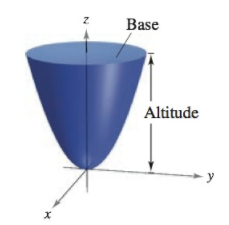Chapter 11, Problem 7PS

Chapter
Section
Textbook Problem

# Volume(a) Find the volume of the solid bounded below by the paraboloid z = x 2 + y 2 and above by the plane z = 1. (b) Find the volume of the solid bounded below by the elliptic paraboloid z = x 2 a 2 + y 2 b 2 and above by the plane z = k , where k > 0. (c) Show that the volume of the solid in part (b) is equal to one-half the product of the area of the base times the altitude, as shown in the figure.(a)

To determine

To calculate: The volume of the solid bounded below by theparaboloid z=x2+y2 and above the plane z=1.

Explanation

Given: The paraboloid z=x2+y2 and the plane z=1

Formula used: The area is found by solving the integral within the limits.

abf(x)dx=f(b)f(a)

Calculation: The volume of solid bounded by the paraboloid z=x2+y2 and above the plane z=1 is given by V=πA.

It is known that if the solid around z-axis, then

x2+y2=[r(z)]2

Comparing with the given equation of paraboloid z=x2+y2:

[r(z)]2=z<

(b)

To determine

To calculate: The volume of the solid bounded below by the elliptical paraboloid z=x2a2+y2b2 and above the plane z=k.

(c)

To determine

To prove: The volume in part (b) is equal to half the product of area of the base, times the altitude.

### Still sussing out bartleby?

Check out a sample textbook solution.

See a sample solution

#### The Solution to Your Study Problems

Bartleby provides explanations to thousands of textbook problems written by our experts, many with advanced degrees!

Get Started